# OliNo

Renewable Energy

## Cor de Ruijter Led light bulb 5 mm leds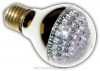Cor de Ruijter presents an led light bulb with 5 mm leds. It has the form of a light bulb. This lamp is in an experimental phase and the sample was a proto sample. This phase is used to confirm the applicability of a unique concept of this lamp: the used transformer in this lamp is changed by a patented circuit. Advantages according to the producer: easier design bringing a cheaper production process, lower energy usage and longer lifetime because a transformer is not used.

This article shows the measurement results. Many parameters are also found in the Eulumdat file.

See this overview for a comparison with other light bulbs.

### Summary measurement data

parameter meas. result remark
Color temperature 9068 K Cold white
Luminous intensity Iv 562 Cd Measured straight underneath the lamp
Beam angle 18 deg Valid for all C-angles as there is symmetry along the 1st axis.
Power P 2.5 W
Power Factor 0.56 For every 1 kWh net power consumed, there has been 1.5 kVAhr for reactive power.
Luminous flux 128 Lm
Luminous efficacy 51 Lm/W
CRI_Ra 81 Color Rendering Index.
Coordinates chromaticity diagram x=0.2913 and y=0.2836
Fitting E27
PAR-value 6.1 μMol/s/m2 The number of photons seen by an average plant when it is lit by the light of this light bulb. Value valid at 1 m distance from light bulb.
S/P ratio 2.3 This factor indicates the amount of times more efficient the light of this light bulb is perceived under scotopic circumstances (ow environmental light level).
D x H external dimensions 60 x 101 mm External dimensions of the lamp (D = diameter).
D x H luminous area 60 x 30 mm Dimensions of the luminous area (used in Eulumdat file). This is equal to the surface of the area on which the leds are mounted.
General remarks The ambient temperature during the whole set of measurements was 23.5-25.5 deg C. The temperature of the lamp gets about 18 degrees hotter than ambient.
Warm up effect: during the warm up time the illuminance decreases with 14 %.

Voltage dependency: the power consumption and illuminance vary linearly on the voltage when it is varied from 200 – 250 V.

Measurement report (PDF)Eulumdat fileRight click on icon and save the file.

### Overview table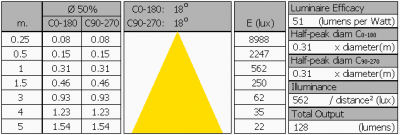The overview table is explained on the OliNo website. Please note that this overview table makes use of calculations, use this data with care as explained on the OliNo site. E (lux) values are not accurate, when within 5 x 60 mm = 300 mm. Within this distance from the lamp, the measured lux values willl be less than the computed values in this overview as the measurements are then within the near field of the lamp.

### Eulumdat light diagram

This light diagram below comes from the program Qlumedit, that extracts these diagrams from an Eulumdat file. It is explained on the OliNo site.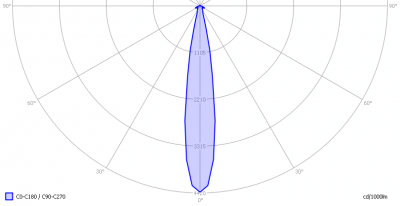The light diagram giving the radiation pattern.

It indicates the luminous intensity around the light bulb. The directions C90-C270 and C0-C180 give the same result as there is a symmetry along the 1st axis.

### Illuminance Ev at 1 m distance, or luminous intensity Iv

Herewith the plot of the averaged luminous intensity Iv as a function of the inclination angle with the light bulb.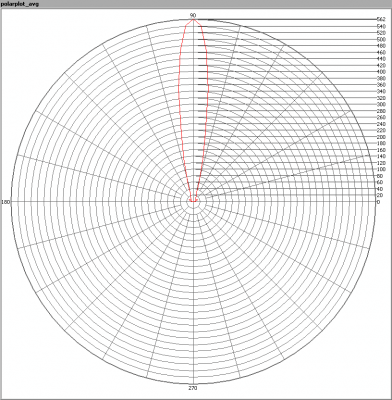The radiation pattern of the light bulb.

This radiation pattern is the average of the light output of the light diagram given earlier. Also, in this graph the luminous intensity is given in Cd.

These averaged values are used (later) to compute the lumen output.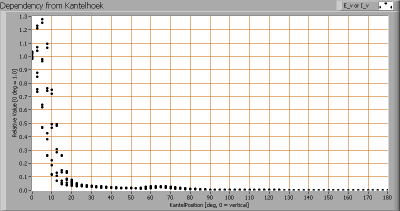Intensity data of every measured turn angle at each inclination angle.

This plot shows per inclination angle the intensity measurement results for each turn angle at that inclination angle. There normally are differences in illuminance values for different turn angles. However for further calculations the averaged values will be used.

When using the average values per inclination angle, the beam angle can be computed, being 18º.

### Luminous flux

With the averaged illuminance data at 1 m distance, taken from the graph showing the averaged radiation pattern, it is possible to compute the luminous flux.

The result of this computation for this light spot is a luminous flux of 128 Lm.

### Luminous efficacy

The luminous flux being 128 Lm, and the power of the light bulb being 2.5 W, yields a luminous efficacy of 51 Lm/W.

### Electrical properties

A power factor of 0.56 means that for every 1 kWh net power consumed, a reactive component of 1.5 kVAr was needed.

 Lamp voltage 230 VAC Lamp current 20 mA Power P 2.5 W Apparent power S 4.5 VA Power factor 0.56

Of this light bulb the voltage across ad the resulting current through it are measured and graphed. See the OliNo site how this is obtained.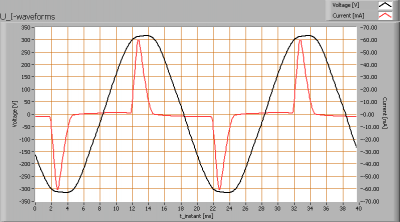Voltage across and current through the lightbulb

The higher harmonics of the current are computed.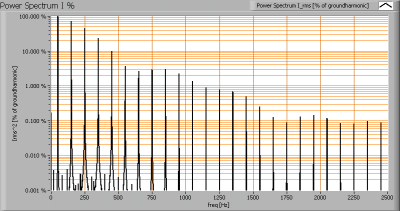The squared amplitudes of the higher harmonics of the current through the lamp

There are no limits for the harmonics for led lighting equipment <= 25 W.

The Total Harmonic Distortion of the current is computed as 143 %.

### Temperature measurements lamp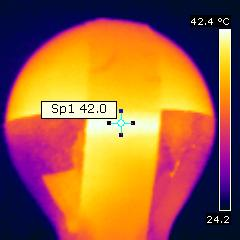Temperature image (overview) of the light bulb

Tape was used on the housing to get a known emissivity.

 status lamp > 2 hours on ambient temperature 23.5 deg C reflected background temperature 23.5 deg C camera Flir B-CAM Western S emissivity 0.95(1) measurement distance 0.15 m IFOVgeometric 0.6 mm NETD (thermal sensitivity) 100 mK

(1) The emissivity is set at 0.95 which is close to the value of the masking tapes used.

The base gets warm-to-the-touch.

### Color temperature and Spectral power distribution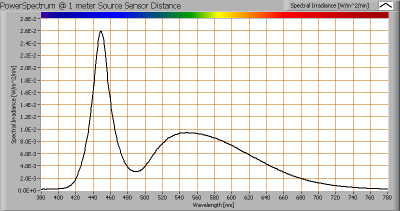The spectral power distribution of this light bulb, energies on y-axis valid at 1 m distance.

The measured color temperature is about 9075 K which is cold white.

This color temperature is measured straight underneath the light bulb. Below a graph showing the color temperature for different inclination angles.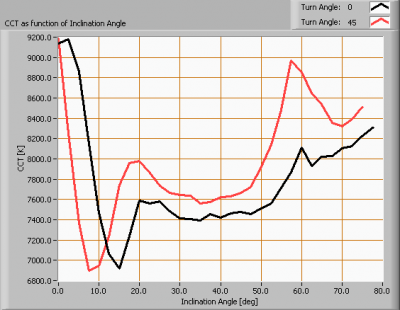Color temperature as a function of inclination angle.

The measurement of CCT is measured for inclination angles up to 75º, since beyond these angles the illuminance was value low (< 5 lux).

The beam angle is maximally 18º, meaning a 9º inclination angle. In this area the majority of the light is present. The variation in correlated color temperature in this area is 25 %.

### PAR value and PAR spectrum

To make a statement how well the light of this light bulb is for growing plants, the PAR-area needs to be determined. See the OliNo website how this all is determined and the explanation of the graph.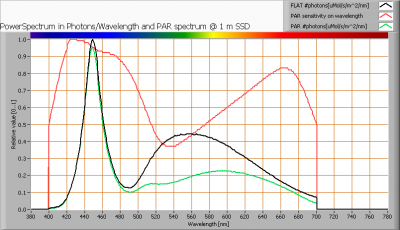The photon spectrum, then the sensitivity curve and as result the final PAR spectrum of the light of this light bulb

parameter value unit
PAR-number 6.1 μMol/s/m²

The PAR efficiency is 69 % (valid for the PAR wave length range of 400 – 700 nm). So maximally 69 % of the total of photons in the light is effectively used by the average plant (since the plant might not take 100 % of the photons at the frequency where its relative sensitivity is 100 %).

### S/P ratio

The S/P ratio and measurement is explained on the OliNo website. Here the results are given.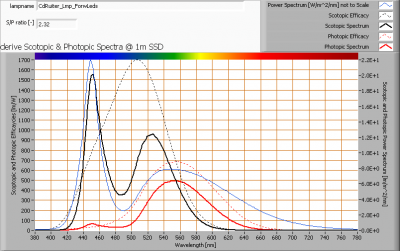The power spectrum, sensitivity curves and resulting scotopic and photopic spectra (spectra energy content defined at 1 m distance).

The S/P ratio is 2.3.

More info on S/P ratio can be found on the OliNo website.

### Chromaticity diagram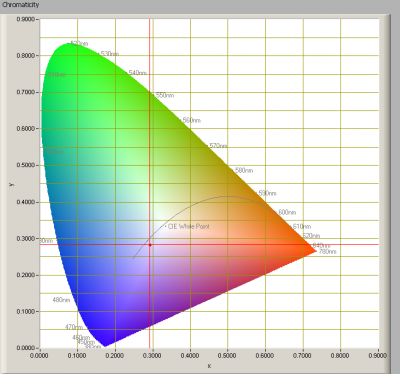The chromaticity space and the position of the lamp’s color coordinates in it.

The light coming from this lamp is at a distance from the Planckian Locus (the black path in the graph).

Its coordinates are x=0.2913 and y=0.2836.

### Color Rendering Index (CRI) or also Ra

Herewith the image showing the CRI as well as how well different colors are represented (rendered). The higher the number, the better the resemblance with the color when a black body radiator would have been used (the sun, or an incandescent lamp). Practical information and also some critics about the CRI can be found on the OliNo website.

Each color has an index Rx, and the first 8 indexes (R1 .. R8) are averaged to compute the Ra which is equivalent to the CRI.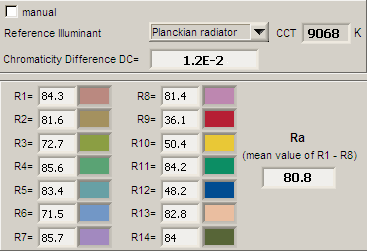CRI of the light of this lightbulb.

The value of 81 is lower than 80 which is considered a minimum value for indoor usage.

Note: the chromaticity difference is 0.012 indicates the distance to the Planckian Locus. There is no norm yet that states what the max deviation from white light is allowed to be.

### Voltage dependency

The dependency of a number of lamp parameters on the lamp voltage is determined. For this, the lamp voltage has been varied and its effect on the following light bulb parameters measured: illuminance E_v [lx], the lamppower P [W], the (Correlated) Color Temperature [K] and the luminous efficacy [Lm/W].Lamp voltage dependencies of certain light bulb parameters, where the value at 230 V is taken as 100 %.

The illuminance and consumed power do vary in a linear way when the voltage is varied.

When the voltage at 230 V varies with + and – 5 V, then the illuminance varies ≈ 12 %, so when abrupt voltage changes occur this effect is visible in the illuminance output.

### Warm up effects

After switch on of a cold lamp, the effect of heating up of the lamp is measured on illuminance E_v [lx], color temperature CT or correlated color temperature CCT [K], the lamppower P [W] and the luminous efficacy [lm/W].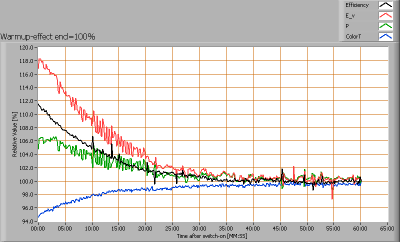Effect of warming up on different light bulb parameters. At top the 100 % level is put at begin, and at bottom at the end.

The warm up time is about 30 minutes. During that time the illuminance decreases with ≈ 14 %.